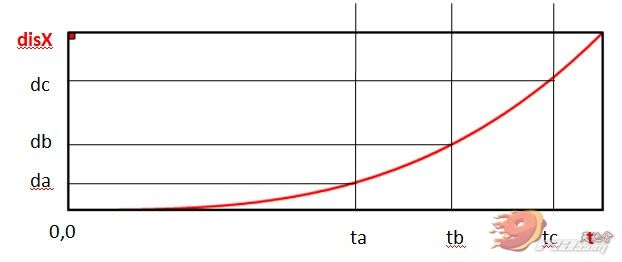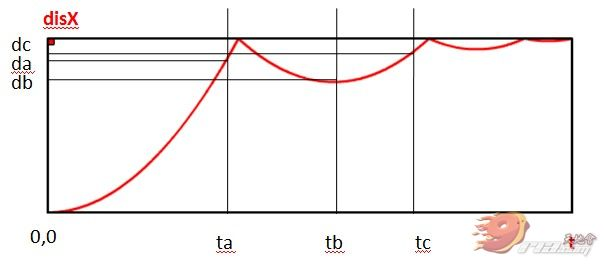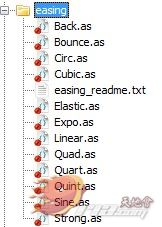# 【转】原来Tween缓冲是这么回事

### Tween缓冲

Tween缓冲大家应该都不陌生，说白了就是从一起始位置逐渐移动到目标位置的过程，这个过程可以是加速移动，也可以是减速移动，这些不同的缓动方式就是Tween的各种ease。

### Tween算法• time：缓动经历过的时间
• beforeMove：起始位置
• changeDistance：起始位置与目标位置的距离,也就是距离上的一个变化量
• duration：我们要求对象从起始位置移动到目标位置所需的时间，也就是缓动的总时长

``````1pos = changeDistance * ( time/duration ) + beforeMove
``````

`````` 1package gs.easing {
2public class Linear {
3	public static function easeNone (t:Number, b:Number, c:Number, d:Number):Number
4	{
5		return c*t/d + b;
6	}
7	public static function easeIn (t:Number, b:Number, c:Number, d:Number):Number
8	{
9		return c*t/d + b;
10	}
11	public static function easeOut (t:Number, b:Number, c:Number, d:Number):Number
12	{
13		return c*t/d + b;
14	}
15	public static function easeInOut (t:Number, b:Number, c:Number, d:Number):Number
16	{
17		return c*t/d + b;
18	}
19}
20}
``````

``````1factor = Linear.easeIn ( t,0,1,d);
2object.para = changeValue * factor + beforeValue;
``````